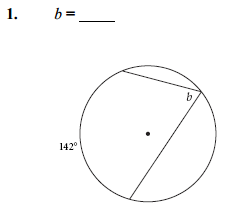# 9.3 Arcs and Angles 1

## Inscribed angle

An inscribed angle is an angle formed by two chords in a circle which have a common endpoint. This common endpoint forms the vertex - C - of the inscribed angle. The other two endpoints - B and D - define what we call an intercepted arc on the circle. ... The minor arc is the smaller of the two arcs (arc BD) while the major arc is the bigger (arc BCD).

## Investigation 1: Inscribed Angle Properties In this investigation you’ll discover a relationship between an inscribed angle and the arc it intercepts.

1. Drag point D (but not past points C or B) and look for a relationship between the arc measure and the measure of the inscribed angle. Make a conjecture (Inscribed Angle Conjecture). 2. Drag point C. As long as you don’t drag it past points B and D, the measurements don’t change. Is your computer broken? Well, dragging point C doesn’t do anything to the arc. What does that mean for all the inscribed angles that intercept that arc? If you’re not sure, construct and measure another inscribed angle that intercepts BDX. Write a conjecture about inscribed angles that intercept the same arc (Inscribed Angles Intercepting Arcs Conjecture).

Inscribed Angle Conjecture The measure of an angle inscribed in a circle is ___________________________.

Check all that applyCheck all that apply